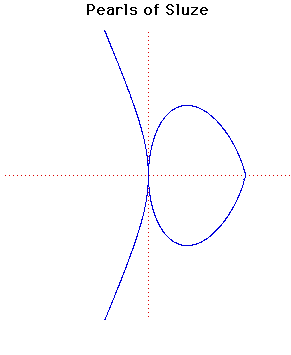# Pearls of SluzeCartesian equation:
yn = k(a - x)pxm

The curves with the equation given above, where n, p and m are integers, were studied by de Sluze between 1657 and 1698.

The name Pearls of Sluze was given to these curves by Blaise Pascal.

The particular curves drawn above have

n = 4, k = 2, a = 4, p = 3, m = 2.

JOC/EFR/BS January 1997